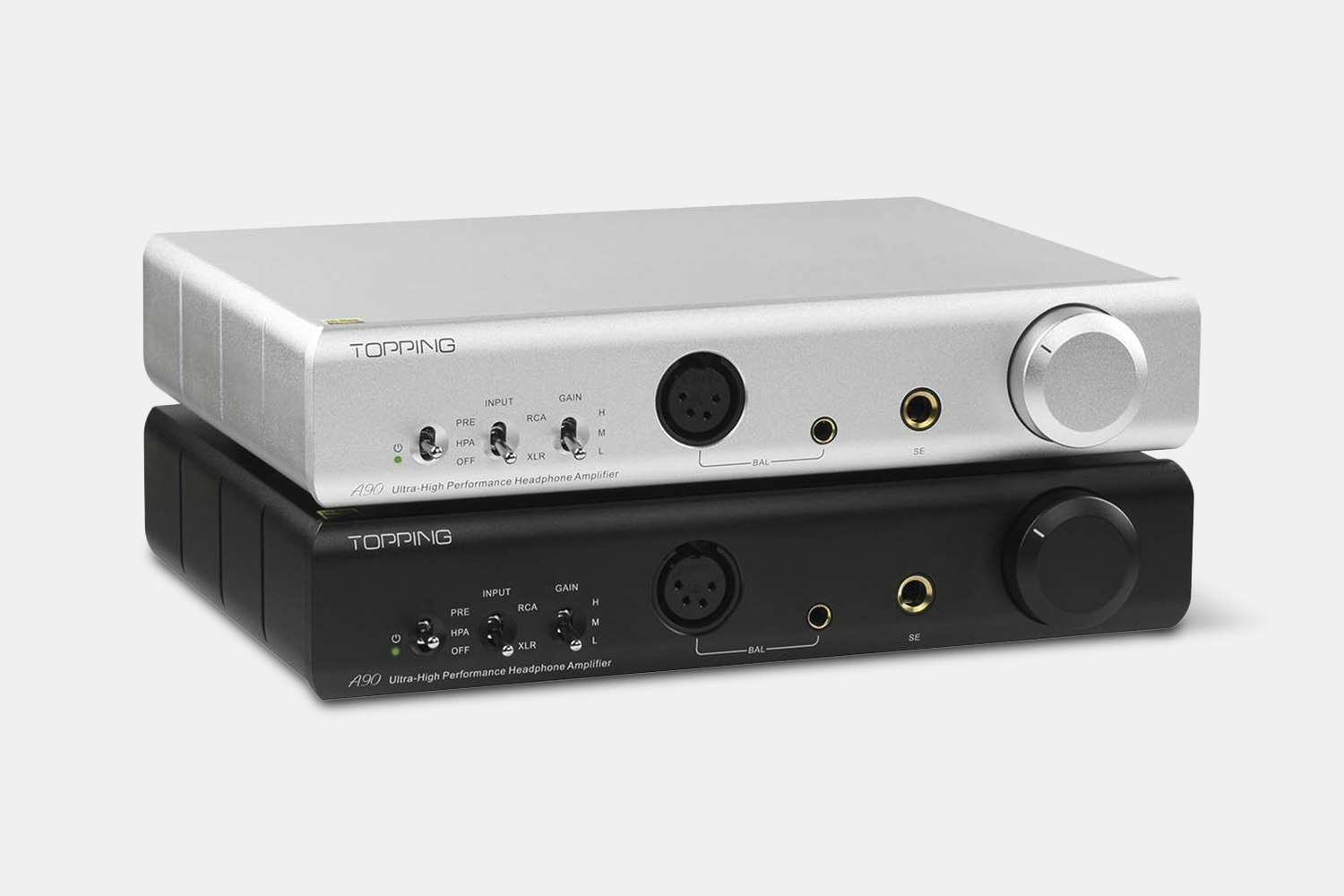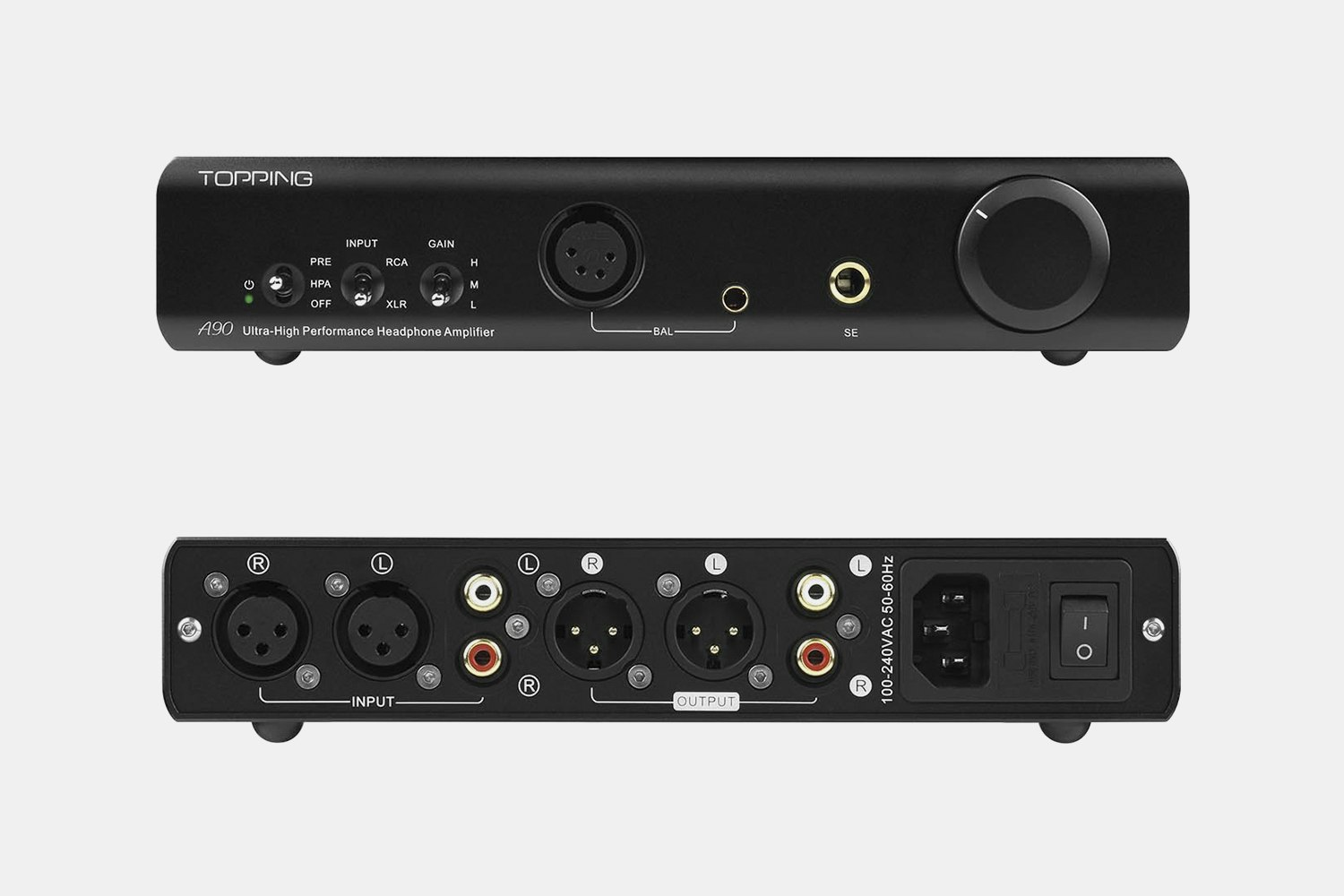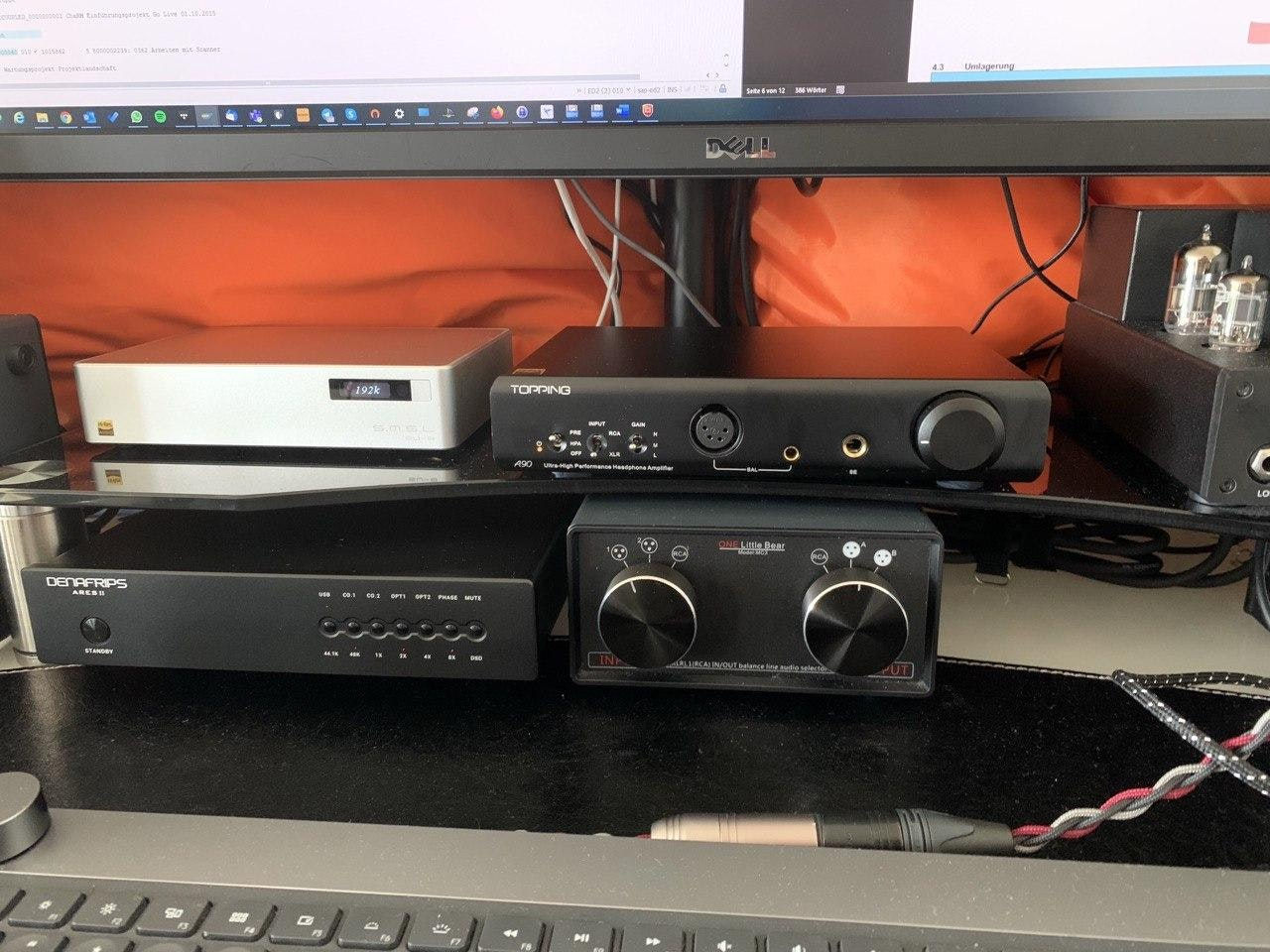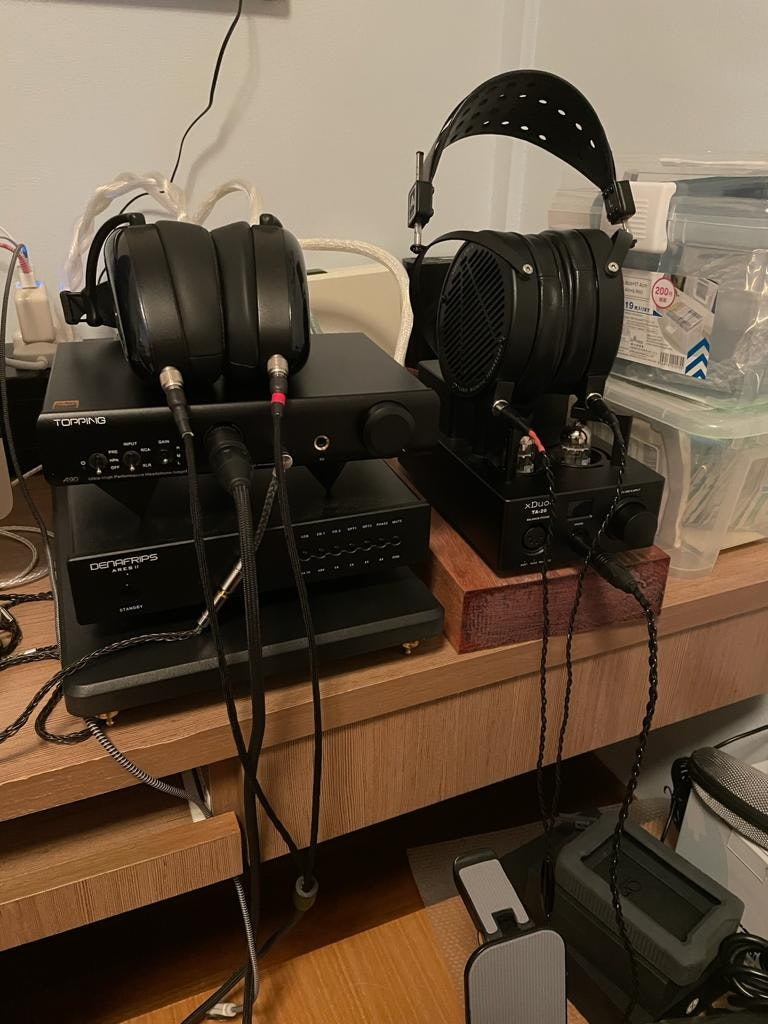bookmark_border
Where's the price?
To negotiate the best possible price for our customers, we agree to hide prices prior to logging in.
3 Sold
Product Description
At the top of Topping’s highly regarded A-series, the A90 is a headphone amplifier with few rivals. Capable of functioning as both a headphone amp and a preamp, this flagship unit is outfitted with NFCA (Nested Feedback Composite Amplifier) modules, which work together with ultra-high-gain feedback technology for impressive AC and DC control Read More

At the top of Topping’s highly regarded A-series, the A90 is a headphone amplifier with few rivals. Capable of functioning as both a headphone amp and a preamp, this flagship unit is outfitted with NFCA (Nested Feedback Composite Amplifier) modules, which work together with ultra-high-gain feedback technology for impressive AC and DC control. Its high-current capabilities make it a great choice for low-impedance headphones, while its 145-decibel dynamic range and 0.2uV noise rating are ideal for more sensitive IEMs. Other notable specs include an output impedance less than 0.1 ohms, a THD+N rating less than 0.00006 percent, and a two-channel 7,200-milliwatt maximum power output. Choose from three gain settings and a 4-pin XLR, 4.4-millimeter balanced, or 6.35-millimeter single-ended port. Switch to preamp mode and connect the A90 to a power amp or DAC such as the D90 to drive active speakers.

Note: This run is limited to the A90 in black with a US plug.Model
Specs

Topping A90 (Headphone Amp) – Single-Ended Input / Output

• THD + N at 1 kHz (A-weighted): < 0.00007% at 500 mW (32 ohms) / < 0.00006% at 80 mW (300 ohms)
• THD at 20 Hz – 20 kHz, 90kBW: < 0.0004% at 500 mW (32 ohms) / < 0.0001% at 80 mW (300 ohms)
• SNR at maximum output 1 kHz (A-weighted): 141 dB
• Dynamic range at 1 kHz (A-weighted): 141 dB
• Frequency response:  20 Hz – 40 kHz (± 0.05 dB)
• Output level: 9 Vpp at G = L, 25 Vpp at G = M, 25 Vpp at G = H
• AP measured noise level (A-weighted): < 0.7 uVrms at G = L, < 0.7 uVrms at G = M, < 0.9 uVrms at G = H
• Actual noise level (A-weighted):< 0.2 uVrms at G = L, < 0.2 uVrms at G = M, < 0.6 uVrms at G = H
• Channel crosstalk at 1 kHz: -106 dB
• IMD CCIF at 18 kHz + 19 kHz: -118 dB
• IMD SMPTE at 60 Hz + 7 kHz: -110 dB at SMPTE 4:1 / -115 dB at SMPTE 1:1
• Input sensitivity: < 9.5 Vrms at G = L, < 8.8 Vrms at G = M, < 2.9 uVrms at G = H
• Gain: -9.5 dB at G = L, 0 dB at G = M, 9.5 dB at G = H
• Output impedance: < 0.1 ohms
• Output power: 3,300 mW x 2 at 16 ohms (THD + N: < 0.1 %), 2,000 mW x 2 at 32 ohms (THD + N: < 0.1 %), 250 mW x 2 at 300 ohms (THD + N: < 0.1 %), 125 mW x 2 at 600 ohms (THD + N: < 0.1 %)
• Load impedance: > 8 ohms

Topping A90 (Headphone Amp) – Balanced Input / Single-Ended Output

• THD + N at 1 kHz (A-weighted): < 0.00009% at 500 mW (32 ohms) / < 0.00006% at 80 mW (300 ohms)
• THD at 20 Hz – 20 kHz, 90kBW: < 0.0004% at 500 mW (32 ohms) / < 0.0001% at 80 mW (300 ohms)
• SNR at maximum output 1 kHz (A-weighted): 137 dB
• Dynamic range at 1 kHz (A-weighted): 137 dB
• Frequency response:  20 Hz – 40 kHz (± 0.01 dB)
• Output level: 9 Vpp at G = L, 25 Vpp at G = M, 25 Vpp at G = H
• AP measured noise level (A-weighted): < 0.7 uVrms at G = L, < 0.7 uVrms at G = M, < 0.9 uVrms at G = H
• Actual noise level (A-weighted):< 0.2 uVrms at G = L, < 0.2 uVrms at G = M, < 0.6 uVrms at G = H
• Channel crosstalk at 1 kHz: -102 dB
• IMD CCIF at 18 kHz + 19 kHz: -118 dB
• IMD SMPTE at 60 Hz + 7 kHz: -110 dB at SMPTE 4:1 / -115 dB at SMPTE 1:1
• Input sensitivity: < 9.5 Vrms at G = L, < 8.8 Vrms at G = M, < 2.9 uVrms at G = H
• Gain: -9.5 dB at G = L, 0 dB at G = M, 9.5 dB at G = H
• Output impedance: < 0.1 ohms
• Output power: 3,300 mW x 2 at 16 ohms (THD + N: < 0.1 %), 2,000 mW x 2 at 32 ohms (THD + N: < 0.1 %), 250 mW x 2 at 300 ohms (THD + N: < 0.1 %), 125 mW x 2 at 600 ohms (THD + N: < 0.1 %)
• Load impedance: > 8 ohms

Topping A90 (Headphone Amp) – Single-Ended Input / Balanced Output

• THD + N at 1 kHz (A-weighted): < 0.00007% at 500 mW (32 ohms) / < 0.00006% at 80 mW (300 ohms)
• THD at 20 Hz – 20 kHz, 90kBW: < 0.0004% at 500 mW (32 ohms) / < 0.0001% at 80 mW (300 ohms)
• SNR at maximum output 1 kHz (A-weighted): 145 dB
• Dynamic range at 1 kHz (A-weighted): 145 dB
• Frequency response:  20 Hz – 40 kHz (± 0.05 dB)
• Output level: 18 Vpp at G = L, 49 Vpp at G = M, 49 Vpp at G = H
• AP measured noise level (A-weighted): < 0.9 uVrms at G = L, < 0.9 uVrms at G = M, < 1.4 uVrms at G = H
• Actual noise level (A-weighted):< 0.6 uVrms at G = L, < 0.6 uVrms at G = M, < 1.3 uVrms at G = H
• Channel crosstalk at 1 kHz: -105 dB
• IMD CCIF at 18 kHz + 19 kHz: -118 dB
• IMD SMPTE at 60 Hz + 7 kHz: -110 dB at SMPTE 4:1 / -115 dB at SMPTE 1:1
• Input sensitivity: < 9.5 Vrms at G = L, < 8.8 Vrms at G = M, < 2.9 uVrms at G = H
• Gain: -3.0 dB at G = L, 6 dB at G = M, 15.5 dB at G = H
• Output impedance: < 0.2 ohms
• Output power: 7,600 mW x 2 at 16 ohms (THD + N: < 0.1 %), 6,400 mW x 2 at 32 ohms (THD + N: < 0.1 %), 1000 mW x 2 at 300 ohms (THD + N: < 0.1 %), 500 mW x 2 at 600 ohms (THD + N: < 0.1 %)
• Load impedance: > 8 ohms

Topping A90 (Headphone Amp) – Balanced Input / Output

• THD + N at 1 kHz (A-weighted): < 0.00009% at 500 mW (32 ohms) / < 0.00006% at 80 mW (300 ohms)
• THD at 20 Hz – 20 kHz, 90kBW: < 0.0004% at 500 mW (32 ohms) / < 0.0001% at 80 mW (300 ohms)
• SNR at maximum output 1 kHz (A-weighted): 138 dB
• Dynamic range at 1 kHz (A-weighted): 138 dB
• Frequency response:  20 Hz – 40 kHz (± 0.01 dB)
• Output level: 18 Vpp at G = L, 49 Vpp at G = M, 49 Vpp at G = H
• AP measured noise level (A-weighted): < 0.9 uVrms at G = L, < 0.9 uVrms at G = M, < 1.4 uVrms at G = H
• Actual noise level (A-weighted):< 0.6 uVrms at G = L, < 0.6 uVrms at G = M, < 1.3 uVrms at G = H
• Channel crosstalk at 1 kHz: -102 dB
• IMD CCIF at 18 kHz + 19 kHz: -118 dB
• IMD SMPTE at 60 Hz + 7 kHz: -110 dB at SMPTE 4:1 / -115 dB at SMPTE 1:1
• Input sensitivity: < 9.5 Vrms at G = L, < 8.8 Vrms at G = M, < 2.9 uVrms at G = H
• Gain: -3.0 dB at G = L, 6 dB at G = M, 15.5 dB at G = H
• Output impedance: < 0.2 ohms
• Output power: 7,600 mW x 2 at 16 ohms (THD + N: < 0.1 %), 6,400 mW x 2 at 32 ohms (THD + N: < 0.1 %), 1000 mW x 2 at 300 ohms (THD + N: < 0.1 %), 500 mW x 2 at 600 ohms (THD + N: < 0.1 %)
• Load impedance: > 8 ohms

Topping (A90 Preamp) – Single-Ended Input / Output

• THD + N at 1 kHz (A-weighted): < 0.00006%
• THD at 20 Hz – 20 kHz, 90kBW: < 0.0001%
• SNR at 1 kHz (A-weighted): 141 dB
• Dynamic range at 1 kHz (A-weighted): 141 dB
• Frequency response:  20 Hz – 40 kHz (± 0.05 dB)
• Output level: 9 Vpp at G = L, 25 Vpp at G = M, 25 Vpp at G = H
• AP measured noise level (A-weighted): < 0.7 uVrms at G = L, < 0.7 uVrms at G = M, < 0.9 uVrms at G = H
• Actual noise level (A-weighted):< 0.2 uVrms at G = L, < 0.2 uVrms at G = M, < 0.6 uVrms at G = H
• Channel crosstalk at 1 kHz: -106 dB
• IMD CCIF at 18 kHz + 19 kHz: -118 dB
• IMD SMPTE at 60 Hz + 7 kHz: -110 dB at SMPTE 4:1 / -115 dB at SMPTE 1:1
• Input sensitivity: < 9.5 Vrms at G = L, < 8.8 Vrms at G = M, < 2.9 uVrms at G = H
• Gain: -9.5 dB at G = L, 0 dB at G = M, 9.5 dB at G = H
• Input impedance: 20 ohms

Topping (A90 Preamp) – Balanced Input / Single-Ended Output

• THD + N at 1 kHz (A-weighted): < 0.00006%
• THD at 20 Hz – 20 kHz, 90kBW: < 0.0001%
• SNR at 1 kHz (A-weighted): 137 dB
• Dynamic range at 1 kHz (A-weighted): 137 dB
• Frequency response:  20 Hz – 40 kHz (± 0.1 dB)
• Output level: 9 Vpp at G = L, 25 Vpp at G = M, 25 Vpp at G = H
• AP measured noise level (A-weighted): < 0.7 uVrms at G = L, < 0.7 uVrms at G = M, < 0.9 uVrms at G = H
• Actual noise level (A-weighted):< 0.2 uVrms at G = L, < 0.2 uVrms at G = M, < 0.6 uVrms at G = H
• Channel crosstalk at 1 kHz: -102 dB
• IMD CCIF at 18 kHz + 19 kHz: -118 dB
• IMD SMPTE at 60 Hz + 7 kHz: -110 dB at SMPTE 4:1 / -115 dB at SMPTE 1:1
• Input sensitivity: < 9.5 Vrms at G = L, < 8.8 Vrms at G = M, < 2.9 uVrms at G = H
• Gain: -9.5 dB at G = L, 0 dB at G = M, 9.5 dB at G = H
• Input impedance: 20 ohms

Topping (A90 Preamp) – Single-Ended Input / Balanced Output

• THD + N at 1 kHz (A-weighted): < 0.00006%
• THD at 20 Hz – 20 kHz, 90kBW: < 0.0001%
• SNR at 1 kHz (A-weighted): 145 dB
• Dynamic range at 1 kHz (A-weighted): 145 dB
• Frequency response:  20 Hz – 40 kHz (± 0.05 dB)
• Output level: 18 Vpp at G = L, 49 Vpp at G = M, 49 Vpp at G = H
• AP measured noise level (A-weighted): < 0.9 uVrms at G = L, < 0.9 uVrms at G = M, < 1.4 uVrms at G = H
• Actual noise level (A-weighted):< 0.6 uVrms at G = L, < 0.6 uVrms at G = M, < 1.3 uVrms at G = H
• Channel crosstalk at 1 kHz: -105 dB
• IMD CCIF at 18 kHz + 19 kHz: -118 dB
• IMD SMPTE at 60 Hz + 7 kHz: -110 dB at SMPTE 4:1 / -115 dB at SMPTE 1:1
• Input sensitivity: < 9.5 Vrms at G = L, < 8.8 Vrms at G = M, < 2.9 uVrms at G = H
• Gain: -3.5 dB at G = L, 6.0 dB at G = M, 15.5 dB at G = H
• Input impedance: 40 ohms

Topping (A90 Preamp) – Balanced Input / Output

• THD + N at 1 kHz (A-weighted): < 0.00006%
• THD at 20 Hz – 20 kHz, 90kBW: < 0.0001%
• SNR at 1 kHz (A-weighted): 138 dB
• Dynamic range at 1 kHz (A-weighted): 138 dB
• Frequency response:  20 Hz – 40 kHz (± 0.1 dB)
• Output level: 18 Vpp at G = L, 49 Vpp at G = M, 49 Vpp at G = H
• AP measured noise level (A-weighted): < 0.9 uVrms at G = L, < 0.9 uVrms at G = M, < 1.4 uVrms at G = H
• Actual noise level (A-weighted):< 0.6 uVrms at G = L, < 0.6 uVrms at G = M, < 1.3 uVrms at G = H
• Channel crosstalk at 1 kHz: -102 dB
• IMD CCIF at 18 kHz + 19 kHz: -118 dB
• IMD SMPTE at 60 Hz + 7 kHz: -110 dB at SMPTE 4:1 / -115 dB at SMPTE 1:1
• Input sensitivity: < 9.5 Vrms at G = L, < 8.8 Vrms at G = M, < 2.9 uVrms at G = H
• Gain: -3.5 dB at G = L, 6.0 dB at G = M, 15.5 dB at G = H
• Input impedance: 40 ohms
Included
• 3.5 mm to 6.3 mm adapter
• Owner’s manual
• US power plug
Shipping

All orders will be shipped by Drop.

Estimated ship date is Dec 2, 2020 PT.

Payment will be collected at checkout. After this product run ends, orders will be submitted to the vendor up front, making all orders final. Check the discussion for updates on your order.

Top Customer QuestionsThere aren't any questions yet.
Be the first to submit yours!